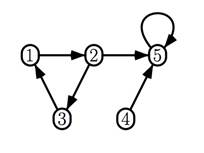﻿ Assignment 2

Assignment 2

UCR - CS 172 –Spring 2020

Instructions: Submit in iLearn by 5/29. This is individual assignment.

Exercise A

1. Compute the first 1 iteration of PageRank scores (d=0.7) of each node in the graph below. Show your work.

2. Write a program (e.g., in Java) to compute the final scores of the nodes and the number of iterations needed to converge, if we use convergence constant epsilon=0.001.

3. If we use personalized PageRank with nodes 1 and 2 in the base set, write the first iteration of the PageRank formulas.Exercise B

Show how MapReduce can be used to efficiently solve the following problem:

Given a collection C of input documents, output a new collection C' of documents, where each document D in C is concatenated with the anchor text of the hyperlinks pointing to D from other documents. For example, if D="hello world", D1 has a hyperlink to D with text "link 1" and D2 has a hyperlink to D with text "link 2", then the output document for D should be D'="hello world link 1 link 2".

Write pseudocode for map and reduce functions.

Full points will be given to efficient solutions.

Exercise C

Consider query Q that has a total of 6 relevant results in the collection, and a search engine that returns results:

r r x x r x x x r,   where x is a relevant result and r is not relevant.

1. Compute Precision-at-5, Recall-at-5, F1-at-5, Average Precision, and DCG-at-5 (assuming relevant results have score 1 and non-relevant 0).

2. Mention an application where higher precision is more important than higher recall and one for the opposite.